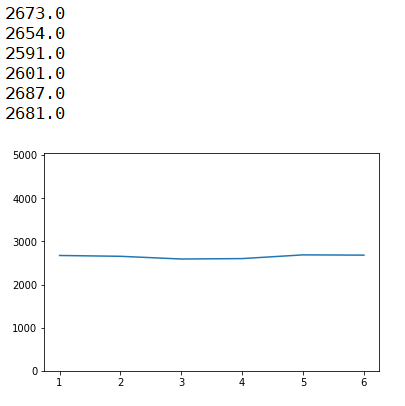# Python program for single dice simulation

Single dice simulation in Python: Here, we are going to learn how to stimulate the occurrence of each dice face i.e. 1, 2, 3, 4, 5, 6?
Submitted by Anuj Singh, on July 31, 2019

Here, we will be stimulating the occurrences of each dice face i.e. 1, 2, 3, 4, 5, 6. Simply we are going to use an inbuilt library called as random to call a random value from given set and thereby we can stimulate the occurrence value by storing the occurrence in the list ls of length 6 representing each face of the dice as ls represents the occurrence of face 5.

```    ls - dice(1)
ls - dice(2)
ls - dice(3)
ls - dice(4)
ls - dice(5)
ls - dice(6)
```

Then using the library pylab, we can plot the value of each occurrence and can stimulate it.

The deviation is clear that each of the faces has an equal almost equal probability of occurrence.

Program:

```import random
import pylab as py

def roll():
return random.choice([1,2,3,4,5,6])

ls = [0,0,0,0,0,0]
chance = [104, 203, 302, 401, 505, 646, 756, 855, 985, 4565, 6565]
for n in chance:
for k in range(n):
scr = roll()
ls[scr-1] = ls[scr-1] + 4/4

py.figure()
py.plot([1,2,3,4,5,6], ls)
py.ylim(0,5040)

for el in ls:
print(el)
```

OutputPreparation